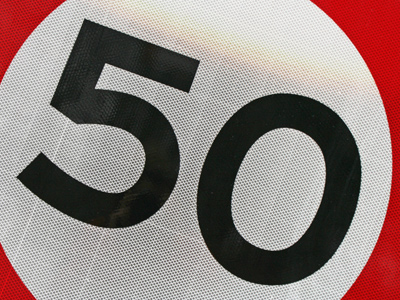To divide a number by 50, divide by 100, then multiply by 2.

# Multiplication and Division 3 (Medium)

Welcome to the third quiz in our Medium section of Eleven Plus maths quizzes on Multiplication and Division. Multiplying or dividing numbers can be difficult sometimes, especially when dealing with large numbers, decimals or fractions. But don’t worry – this quiz is full of helpful handy hints which will make your exams much easier!

The secret to multiplying or dividing difficult numbers is simplification. Here’s an example:

Suppose you were asked to multiply 14 by 17. That sounds quite hard and I doubt many of us can do it in our heads. But if we simplify it then it’s much easier:

14 x 17 = (14 x 10) + (14 x 7) = 140 + 98 = 238

You see how much easier that was? There are lots of other tricks you can use for multiplication and for division. You might even have some of your own! So, try this quiz, read all the helpful comments, and keep playing until you always score a perfect 10 out of 10. Good luck!

1.
What is the correct answer to the given calculation?
138 x 50 = ?
690
6,900
69,000
690,000
To multiply a number by 50: first divide by 2, then move the digits 2 places to the left:
138 × 50 = (138÷ 2) x 100 = 69 x 100. Move the d.p. 2 places to the right gives 6,900
2.
What is the correct answer to the given calculation?
34 x 110 = ?
364
374
3,640
3,740
To multiply a number by 110, first multiply by 100, then multiply by 10 and just add your two answers together:
34 x 110 = (34 x 100) + (34 x 10) = 3,400 = 340 = 3,740
3.
What is the correct answer to the given calculation?
14.72 ÷ 5 = ?
2.875
2.894
2.972
2.944
To divide a number by 5: first multiply by 2 and then move the digits to the right:
14.72 ÷ 5 = (14.72 × 2) ÷ 10 = 29.44 ÷ 10 . Move the d.p. 1 place to the left gives 2.944
4.
What is the correct answer to the given calculation?
3,846 ÷ 200 = ?
19.23
38.46
192.3
384.6
To divide a number by 200, first divide by 2 then divide your answer by 100
3,846 ÷ 2 = 1,923 ÷ 100 = 19.23
5.
What is the correct answer to the given calculation?
18 x 13 = ?
123
234
345
456
To multiply a number by 13, first multiply the number by 10, then multiply it by 3 and finally, add your two answers together:
18 x 13 = (18 x 10) + (18 x 3) = 180 + 54 = 234
6.
What is the correct answer to the given calculation?
406 x 12 = ?
4,836
4,848
4,860
4,872
To multiply a number by 12, first multiply the number by 10, then add twice the original number to it:
406 × 12 = (406 x 10) + (406 x 2) = 4,060 + 812 = 4,872
7.
What is the correct answer to the given calculation?
17 x 90 = ?
1,270
1,450
1,530
1,610
To multiply a number by 90: first multiply the number by 10, then subtract the original number from it, and finally multiply your answer by 10:
17 × 90 = ((17 x 10) - 17) x 10 = (170 - 17) x 10 = 153 x 10 = 1,530
8.
What is the correct answer to the given calculation?
24 x 800 = ?
19,200
1,920
192,000
192
To multiply a number by 800, multiply the number by 2, then by 2 again, and again by 2, and finally by 100:
24 × 800 = (24 x 2 x 2 x 2) x 100 = (48 x 2 x 2) x 100 = (96 x 2) x 100 = 192 x 100 = 19,200
9.
What is the correct answer to the given calculation?
16.5 x 25 = ?
420.5
416.5
412.5
408.5
To multiply a number by 25: multiply by 100, then divide by 4:
16.5 × 25 = (16.5 x 100) ÷ 4 = 1,650 ÷ 4 = 412.5
10.
What is the correct answer to the given calculation?
1,275 ÷ 50 = ?
2.55
25.5
255
0.255
To divide a number by 50: divide by 100, then multiply by 2: 1,275 ÷ 50 = (1,275 ÷ 100) x 2 = 12.75 x 2 = 25.5
Author:  Frank Evans